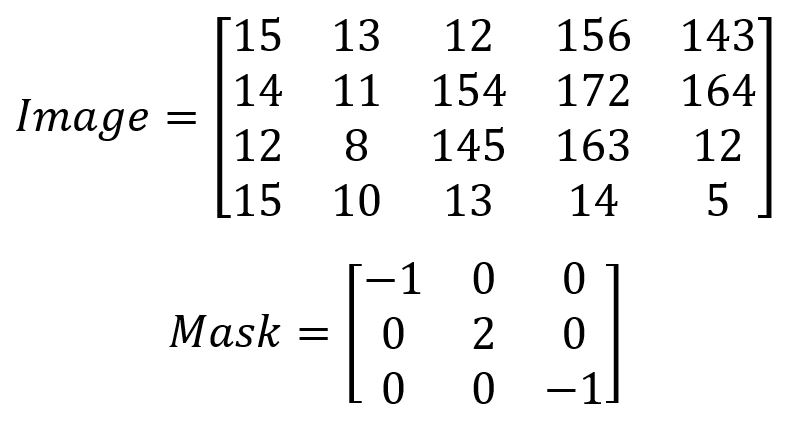Robotics 2
QuizQuestion 7:  Which of the following describes what is meant by 'image segmentation'?
Question 8:  In an 'object matrix', what do the values of the pixels represent?
Question 9:  When we applied image segmentation and masking in Python, what was the purpose of the 'opening' algorithm we used?
Question 10:  The object labeling algorithm we learned in the Image Segmentation video is the same algorithm we used from Open CV in the Python video.  In the Python video, what did we call that algorithm?
Question 1-6: Suppose I have an image as shown here, and I apply the mask as shown.  Fill in the values of the result image.  Some of your numbers might be less than 0 or greater than 255.  That's ok; just enter the values as you calculate them.
Multiplying a large image by a small image to get a result image
Splitting up one large image into smaller image pieces that are easier to process
Identifying the objects in a scene - for example, whether an object is a car or a tree
Identifying which pixels of an image belong to which objects in the scene
The brightness of a particular pixel
The RGB color value of a particular pixel
Which object the pixel belongs to
The size of the overall imageTo reduce the object 'noise'
To increase the brightness of the objects
To increase the size of the objects
To find the locations of the objects in the scene
Morphological operations
The connected pixel algorithm
Opening algorithm
Closing algorithm